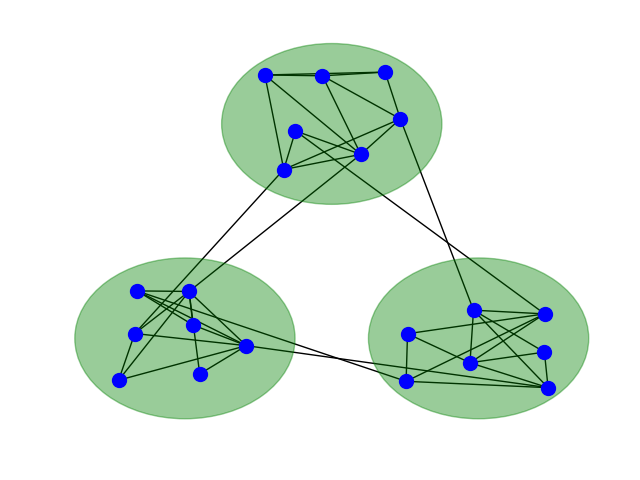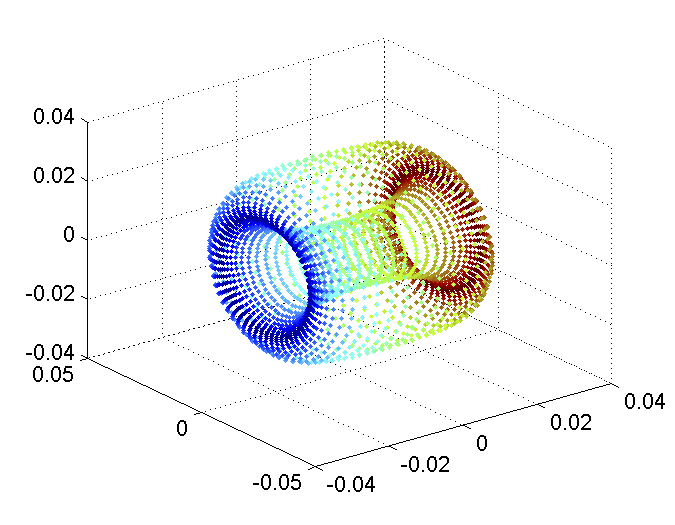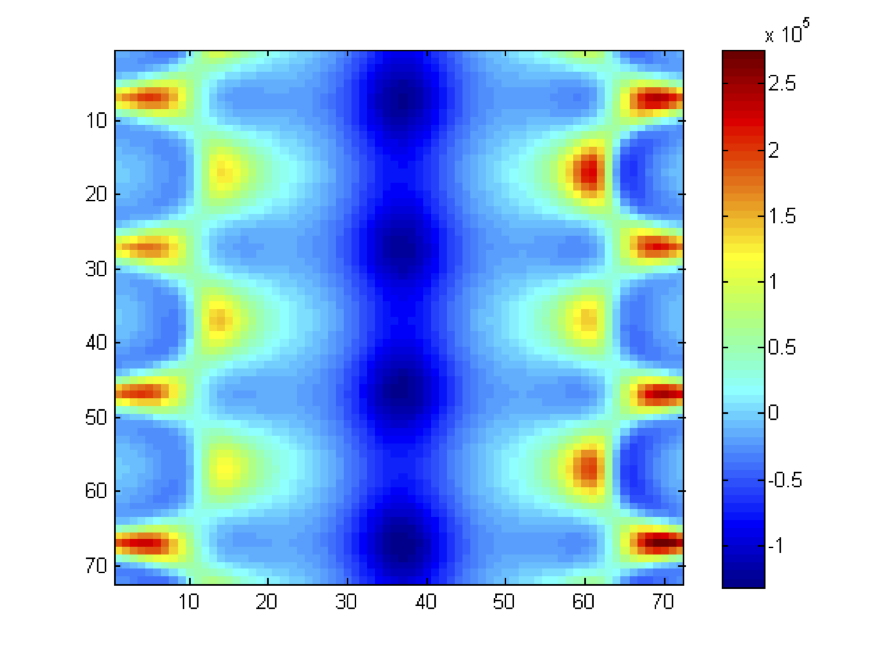UHH > Faculties > MIN-Faculty > Mathematics > Staff > Armin IskeCollaborative Research Project

LD-SODA: Learning-based Data Analysis -

Statistics, Optimization, Dynamics and Approximation

funded by Landesforschungsförderung Hamburg, Hamburger Behörde für Wissenschaft, Forschung und Gleichstellung (BWFG).

## Motivation and Goals

Due to the growing demand for technological progress in digitalization, machine learning methods, such as artificial neural networks, support vector machines or random forests, are of vital importance for relevant applications in science and industry. Learning-based methods work with rather general model assumptions, under which large data sets are being processed. Due to recent advances in measurement engineering and data processing facilities, application data are of rapidly growing size.

This in turn requires more powerful computing facilities to efficiently use learning methods in relevant application areas. To improve the reliability and performance of existing learning methods, more powerful algorithms need to be developed, at the reduction of their potential risks, and for the plausibility and explainability of automated decisions. This in particular needs a better understanding of the underlying mathematical methods, which are fundamental for the development of next generation learning algorithms, on the one hand, and their efficient implementation, on the other hand. While the complexity of machine learning algorithms is rapidly increasing, there is a lack of theoretical understanding of the learning processes.

Fundamental questions, like

• How can we reduce the data complexity?
• How can learning methods describe the dependence of input and output?
• How can we realize optimization processes efficiently?
• How can we understand and control the dynamics of learning processes?
• How can we quantify uncertainties and perturbation of the data?
lead to challenging problems in different mathematical disciplines.

This research project aims at the mathematical analysis of machine learning methods in sufficient width and depth. On the grounds of the mathematical findings, we further aim to improve existing learning methods, or to develop new ones. In this way, we provide a fundamental account to the construction of more advanced learning algorithms The project is a collaboration between scientists from four mathematical disciplines: Stochastics, Optimization, Dynamical Systems and Approximation. The collaboration is split into four projects, which are explained as follows.

## TP-S: Support Vector Machines for statistical inverse problems

Machine learning methods have been successfully applied for data analysis in numerous applications. Thereby, the quality of the procedures is typically measured via the prediction error. Aiming for an approximation of the mapping from input data or features to the output data, a common aspect of learning methods is that they are constructed as a black box. The path from input to output is unspecific and cannot be interpreted.

In this project we apply machine learning methods to inverse problems and study their statistical properties. More precisely, we consider an inverse regression model where the function of interest cannot be directly observed, but is hidden behind some linear operator. While the prediction error corresponds to the forward problem for which learning methods are immediately applicable, the reconstruction of the target function requires to invert the linear operator leading to a possibly ill-posed inverse problem. Such inverse problems are intensively studied in the numerical as well as the statistical literature. However, an analysis of machine learning methods in this context is not well understood due to the previously mentioned black box from which the target function cannot be easily reconstructed. Among the most popular machine learning methods we will focus on kernel methods especially support vector machines.

Project lead: Jun.-Prof. Dr. Mathias Trabs

## TP-O: New Column-Generation Algorithms for Deep Learning

 High-dimensional large data sets from applications require large artificial neural networks. Learning with such networks can be difficult, as the optimization problems required for this are large, nonconvex and nonsmooth. On the other hand, it is often not clear, which network architecture, in particular the number of network layers and components, is the most efficient as this depends on the training data. Too many layers can lead to overfitting and too few layers can deteriorate the approximation quality.Modular neuronal network

In this project we develop new column generation algorithms for simultaneous determination of optimal parameters and architectures of artificial neural networks. Column generation methods have the advantage, that a master problem defined by inner points is generated. This forms the basis for determining a global and robust solution, as well as for the adaptive growth of neural networks and the distribution of weights in network components, such as in modular mixture-of-experts architectures (see figure).

Project lead: Prof. Dr. Ivo Nowak

## TP-D: Dynamics and perturbation growths in learning networks

Artificial neural networks are very successful in classifying images and sounds. Generalizations of their concepts are recently proposed to improve time-series forcasting. In this project we aim on describing the process of training artificial neural networks in terms of a dynamical system approach. Dynamical systems can be characterized through a variety of quantities and methods, as e.g. measures of local and global stability. One important aspect of dynamical systems is the growth of perturbations, and the directions of perturbation grows. An open question is whether the process of training and learning can be improved or accelerated by deliberately perturbing the system, using knowledge about its dynamics.

Project lead: Prof. Dr. Sarah Hallerberg

## TP-A: Kernel-based approximation methods for adaptive data analysis

High performance machine learning methods require efficient algorithms for adaptive data analysis, in particular for very large data sets. In relevant applications, however, training data (of growing sizes) are not only very large, but critical in many different ways, e.g., high-dimensional, scattered, incomplete, or uncertain. A reliable analysis of such critical data requires customized and flexible methods from multivariate approximation. To this end, suitable approximation methods were just recently combined with geometrical projection methods of nonlinear dimensionality reduction (NDR).

In this project, we develop and analyze novel concepts of kernel-based approximation schemes for adaptive data analysis. To this end, we construct non-standard kernel functions, from which we obtain flexible and reliable tools for the analysis of critical data. Moreover, we develop adaptive approximation algorithms of sparse representations of large data sets. In the numerical analysis of the resulting approximation algorithms, particular emphasis is placed on their computational complexity, their accuracy and their numerical stability. The combination between kernel-based approximation and nonlinear dimensionality reduction schemes requires suitable characterizations of topplogical and geometrical invariants, in particular for the purpose of data classification. We finally develop nonlinear projection methods, which are invariant under the identified topological and geometrical properties.NDR: Analysis of topological invariants NDR: Analysis of geometrical invariants

Project lead: Prof. Dr. Armin Iske

## Principal Investigators

Prof. Dr. Sarah Hallerberg (Sprecherin)
Hochschule für Angewandte Wissenschaften Hamburg
Fakultät Technik und Informatik
Department Maschinenbau und Produktion

Prof. Dr. Armin Iske
Universität Hamburg
Fachbereich Mathematik

Prof. Dr. Ivo Nowak
Hochschule für Angewandte Wissenschaften Hamburg
Fakultät Technik und Informatik
Department Maschinenbau und Produktion

Jun.-Prof. Dr. Mathias Trabs
Universität Hamburg
Fachbereich MathematikImpressum 2020-05-08, Gerhard Opfer